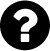## Michael Grünstäudl (Gruenstaeudl), PhD

### Postdoctoral Researcher at the Freie Universität Berlin## Schematic of Plastid Genome in LaTeX

Professional graphics require code

In order to visualize the location of oligonucleotide primers as well as structural rearrangements in plastid genomes, I wrote some Latex code.

\documentclass[margin=10pt]{standalone} \usepackage{tikz} \begin{document} \begin{tikzpicture}[line cap=rect,line width=3pt] \draw[] (0,0) circle [radius=8cm]; % Draw small intervals, label small intervals \foreach \angle [count=\xi, evaluate=\xi as \xx using int(\xi*10)] in {157.5,135,...,-157.5,-180}{ \draw[line width=1.5pt] (\angle:7.8cm) – (\angle:8.2cm); \node[font=\large] at (\angle:9cm) {\xx ,000}; } % Draw quater intervals \foreach \angle [] in {0,-90,-180,-270}{ \draw[line width=3pt] (\angle:7.7cm) – (\angle:8.3cm); } % Draw origin \draw[line width=3pt] (-182:7.7cm) – (-182:8.3cm); \node[font=\large] at (-182:9cm) {origin}; % Draw IRs \draw[line width=5pt,gray] ([shift=(-90:5cm)]5,0) arc (-45:-90:7cm); \draw[line width=5pt,gray] ([shift=(-180:5cm)]0,-5) arc (-135:-180:7cm); % Label IRs \node[font=\large, gray] at (-157.5:6.5cm) {IRa}; \node[font=\large, gray] at (-67.5:6.5cm) {IRb}; \end{tikzpicture} \end{document} 

When compiled, the above code generates the following image:

A thank you goes to some fellow LaTeX Stack Exchange users, who assisted with the above code.

##### Der Beitrag wurde am Monday, den 4. January 2016 um 15:35 Uhr von Michael Grünstäudl veröffentlicht und wurde unter audiovisual, bioinformatics abgelegt. Sie können die Kommentare zu diesem Eintrag durch den RSS 2.0 Feed verfolgen. Sie können einen Kommentar schreiben, oder einen Trackback auf Ihrer Seite einrichten.Hinweis / Hint
Das Captcha kann Kleinbuchstaben, Ziffern und die Sonderzeichzeichen »?!#%&« enthalten.
The captcha could contain lower case, numeric characters and special characters as »!#%&«.